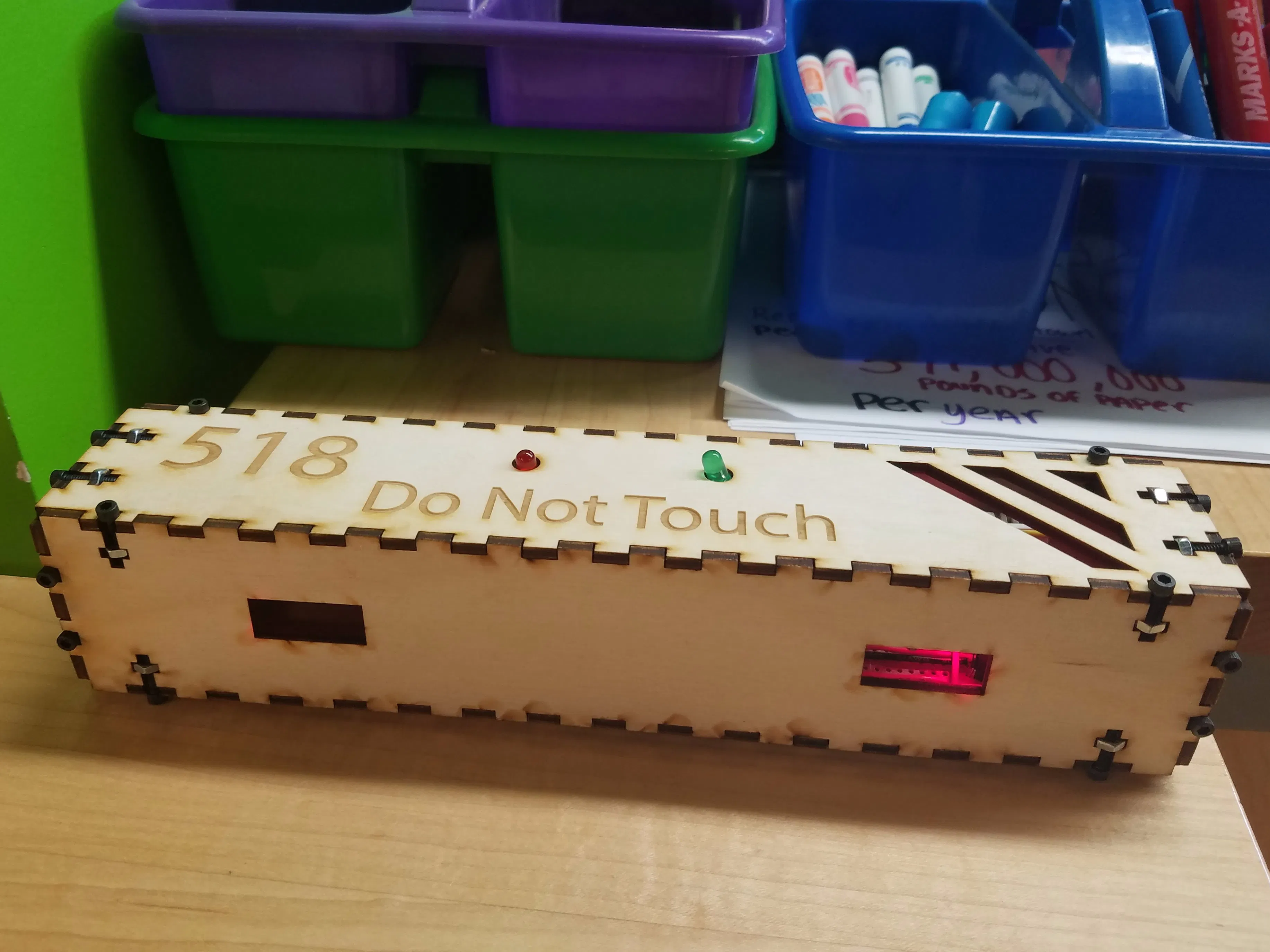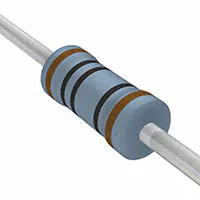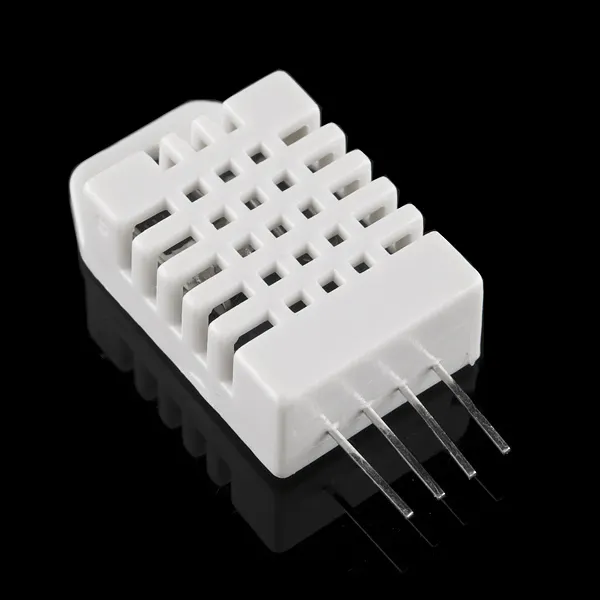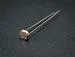Published

# Lane of Things Group 518

A sensor that detects how many people walk in or out of a class room as well as temperature.

IntermediateFull instructions providedOver 2 days460## Things used in this project

### Hardware componentsParticle Photon
×1Resistor 10k ohm
×2Resistor 100 ohm
×1DHT22 Temperature Sensor
×1Photo resistor
×1
×1
×2
 PCB Board
×1
×2

### Hand tools and fabrication machinesSoldering iron (generic)Laser cutter (generic)

## Code

### Lazer tripwire

C/C++
Pushes to Phant server every time someone walks by and google sheets pulling temperature, humidity and whether the door is open or closed.
```// This #include statement was automatically added by the Particle IDE.
#define DHTPIN 2    // what pin we're connected to
#define DHTTYPE DHT22		// DHT 22 (AM2302)

// This #include statement was automatically added by the Particle IDE.
#include <SparkFunPhant.h>

// This #include statement was automatically added by the Particle IDE.
#include <SparkTime.h>

#include "application.h"

SparkTime rtc;
UDP UDPClient;
DHT dht(DHTPIN, DHTTYPE);

unsigned long currentTime;

int entryResist = A0;
int leavingResist = A3;
int door = D6;
int ledLeave = D0;
int ledEnter = D4;
int doorValue;

int period;//records the period number with
//0=Before School // 9=After School // 10=Passing Period
String curclass;
String direc;
String doorOC;
int lastmin;

double temp;
double hum;

const char server[] = "data.sparkfun.com"; // Phant destination server
const char publicKey[] = "bGlXEXz1QLH4XzWaA40X"; // Phant public key - HAS TO BE CHANGED
const char privateKey[] = "Vpol7lAbWPSjaKpq7jJa"; // Phant private key  - HAS TO BE CHANGED
Phant phant(server, publicKey, privateKey); // Create a Phant object

void setup()
{
Particle.variable("temp", temp);
Particle.variable("hum", hum);
Particle.variable("door", doorOC);
rtc.begin(&UDPClient, "time.nist.gov");
rtc.setTimeZone(-6); // gmt offset
pinMode(door,INPUT);
pinMode(entryResist, INPUT);
pinMode(leavingResist, INPUT);
pinMode(DHTPIN, INPUT);
Serial.begin(9600);
currentTime = rtc.now();
lastmin = rtc.minute(currentTime);  //sets time

}

void loop()
{
direc = "None";
/////////////////// Time and Period set ///////////////////

currentTime = rtc.now();
int min = rtc.minute(currentTime);  //sets time
int hour = rtc.hour(currentTime);

if(min != lastmin)
{
if(hour < 8)
{
period = 0;
}

else if(hour == 8 && min <50)
{
period = 1;
}

else if(hour == 8 && (min >= 50 && min < 55))
{
period = 10;
}

else if((hour == 8 && min <= 55) || ( hour == 9 &&  (min >= 0 && min < 45)))
{
period = 2;
}

else if(hour == 9 && (min >= 45 && min < 50))
{
period = 10;
}

else if((hour == 9 && min >= 50) || (hour == 10 && (min >= 0 && min < 40)))
{
period = 3;
}

else if(hour == 10 && (min >= 40 && min < 45))
{
period = 10;
}

else if((hour == 10 && min >= 45) || (hour == 11 && (min >= 0 && min < 35)))
{
period = 4;
}

else if(hour == 11 && (min >= 35 && min < 40))
{
period = 10;
}

else if((hour == 11 && min >= 40) || (hour == 12 && (min >= 0 && min < 30)))
{
period = 5;
}

else if(hour == 12 && (min >= 30 && min < 35))
{
period = 10;
}

else if((hour == 12 && min >= 35) || (hour == 13 && (min >= 0 && min < 25)))
{
period = 6;
}

else if(hour == 13 && (min >= 25 && min < 30))
{
period = 10;
}

else if((hour == 13 && min >= 30) || (hour == 14 && (min >= 0 && min < 20)))
{
period = 7;
}

else if(hour == 14 && (min >= 20 && min < 25))
{
period = 10;
}

else if((hour == 14 && min >= 25) || (hour == 15 && (min >= 0 && min < 15)))
{
period = 8;
}

else if(((hour >= 15) && (min>=15)) || (hour>=16))
{
period = 9;
}

/////////////////// Update Temp & Hum ///////////////////////

double checkHum = dht.getHumidity();
double checkTemp = dht.getTempFarenheit();

if (checkHum >= 0 && checkHum <= 100)
hum = checkHum;

if (checkTemp > 32 && checkTemp < 120)
temp = checkTemp;

lastmin = min;
}

if(period == 0){
curclass = "Before School";
}
if(period == 1){
}
if(period == 2){
curclass = "Engineering Design";
}
if(period == 3){
curclass = "Engineering Design";
}
if(period == 4){
curclass = "No Class";
}
if(period == 5){
curclass = "No Class";
}
if(period == 6){
curclass = "Physics C";
}
if(period == 7){
curclass = "Physics C";
}
if(period == 8){
curclass = "No Class";
}
if(period == 9){
curclass = "After School";
}
if(period == 10){
curclass = "Passing";
}

//////////////////// Door /////////////////////////

if(doorValue == HIGH)
doorOC = "Open";

if(doorValue == LOW)
doorOC = "Closed";

//////////////////// Trip Wire ///////////////////

//Serial.println(enterValue);
//Serial.println(leavingValue);
//Serial.println(period);
//delay(500);

//////////////////// Trigger to Post to Phant ///////////////////

if (enterValue < 3500)
{
direc = "Entering";
digitalWrite(ledEnter, HIGH);
postToPhant();
delay(2000);
digitalWrite(ledEnter, LOW);
}

if(leavingValue < 3500)
{
direc = "Leaving";
digitalWrite(ledLeave, HIGH);
postToPhant();
delay(2000);
digitalWrite(ledLeave, LOW);
}

}

int postToPhant()
{
// Phant requires you to update each and every field before posting,
// make sure all fields defined in the stream are added here.

TCPClient client;
char response;
int i = 0;
int retVal = 0;

if (client.connect(server, 80)) // Connect to the server
{
// Post message to indicate connect success
Serial.println("Posting!");

// phant.post() will return a string formatted as an HTTP POST.
// It'll include all of the field/data values we added before.
// Use client.print() to send that string to the server.
client.print(phant.post());
delay(1000);
// Now we'll do some simple checking to see what (if any) response
// the server gives us.
while (client.available())
{
Serial.print(c);	// Print the response for debugging help.
if (i < 512)
response[i++] = c; // Add character to response string
}
// Search the response string for "200 OK", if that's found the post
// succeeded.
if (strstr(response, "200 OK"))
{
Serial.println("Post success!");
retVal = 1;
}
else if (strstr(response, "400 Bad Request"))
{	// "400 Bad Request" means the Phant POST was formatted incorrectly.
// This most commonly ocurrs because a field is either missing,
// duplicated, or misspelled.
retVal = -1;
}
else
{
// Otherwise we got a response we weren't looking for.
retVal = -2;
}
}
else
{	// If the connection failed, print a message:
Serial.println("connection failed");
retVal = -3;
}
client.stop();	// Close the connection to server.
return retVal;	// Return error (or success) code.
}
```

## Credits

### Xochitl Hubbell-Fox

-1 projects • 0 followers
Past, Present, and Future nerd. College Student. Passionate about fruit.

### Rafal Klecha

-1 projects • 0 followers
Student attending Lane Tech High School in Chicago, Illinois.

### Piotr Ramza

-1 projects • 0 followers
High School student